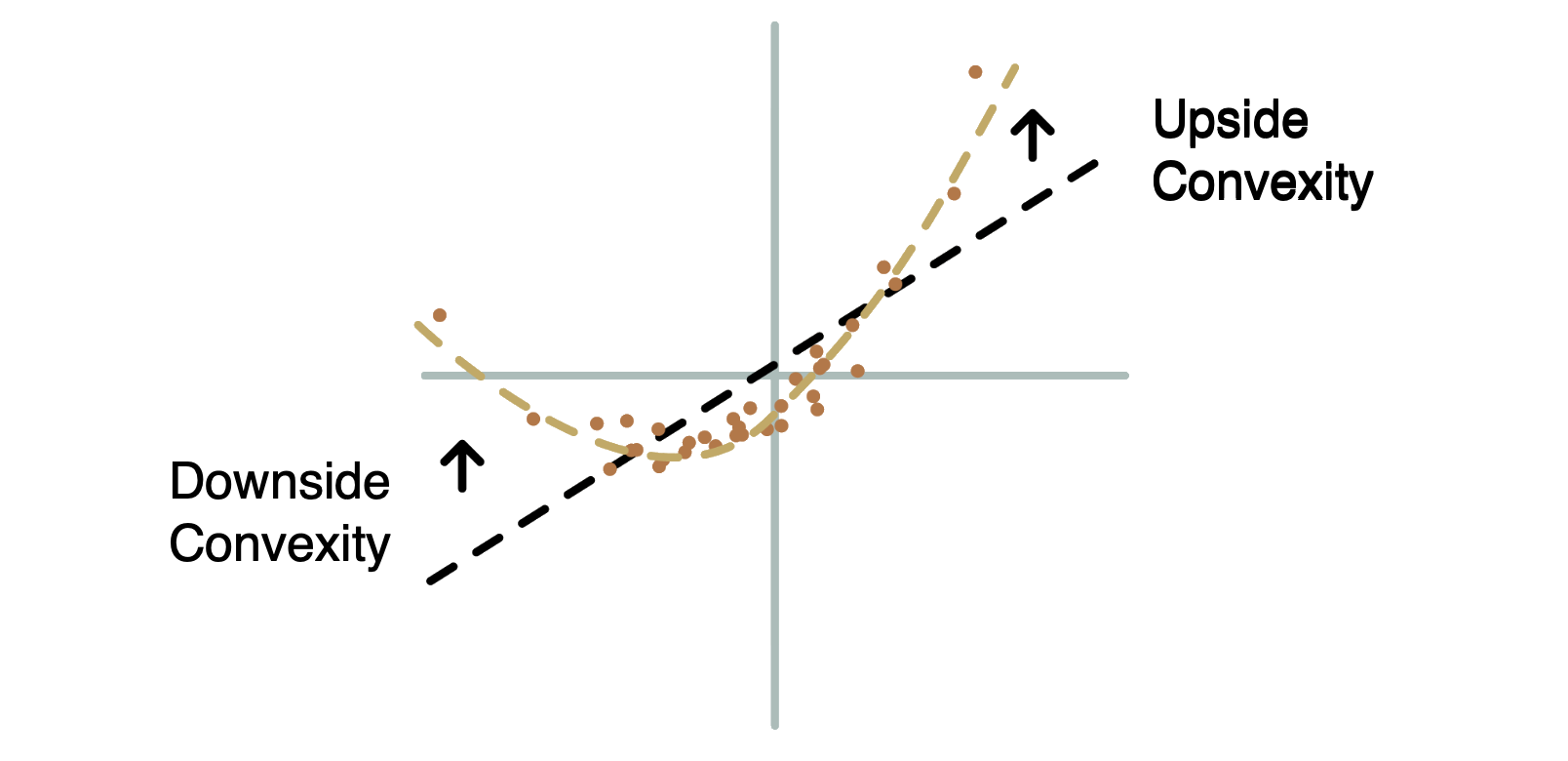Convexity is a concept that originates from mathematics and is widely used in various fields, including finance, optimization, and geometry.

In the context of mathematics, a set is considered convex if, for any two points within the set, the line segment connecting those points lies entirely within the set.

In other words, a set is convex if it contains all the points on the straight line between any two of its points.

In finance and investment, convexity is often used to describe the curvature of the relationship between bond prices and interest rates. It's a measure of how the price of a bond changes in response to fluctuations in interest rates. In simple terms, it indicates how the bond's price sensitivity to interest rate changes varies as interest rates move up or down.

There are two aspects of convexity related to bonds:

1. Positive Convexity: A bond with positive convexity will have a price-yield relationship that is curved upward. This means that as interest rates decrease, the bond's price will increase at an increasing rate. Positive convexity is generally considered favorable for bondholders because it provides some protection against interest rate risk.

2. Negative Convexity: A bond with negative convexity will have a price-yield relationship that is curved downward. This means that as interest rates decrease, the bond's price will increase, but at a decreasing rate. On the other hand, as interest rates increase, the bond's price can decrease more than proportionally. Negative convexity is often associated with callable bonds or mortgage-backed securities.

In options trading, convexity refers to the second-order sensitivity of the option's price to changes in the underlying asset's price. It's a concept used to describe how the option's price changes in response to changes in the underlying asset's price, beyond what can be explained by the first-order sensitivity known as delta.

Delta measures the first-order change in an option's price with respect to a change in the underlying asset's price. However, as the underlying asset's price changes, the relationship between the option's price and the underlying price may not be linear. This is where convexity comes into play.

Convexity accounts for the curvature of the option's price curve with respect to changes in the underlying asset's price. It indicates how the rate of change of delta (which is itself a measure of sensitivity) changes as the underlying asset's price changes.

In the context of options, there are two main aspects of convexity:

1. Positive Convexity: If an option has positive convexity, it means that its delta increases at an increasing rate as the underlying asset's price rises. In other words, positive convexity implies that the option becomes more sensitive to price increases in the underlying asset.

2. Negative Convexity: Conversely, if an option has negative convexity, its delta increases at a decreasing rate as the underlying asset's price rises. This means that the option becomes less sensitive to price increases in the underlying asset.Convexity is particularly relevant when considering large changes in the underlying asset's price. It helps options traders and investors understand the potential non-linear behavior of options prices and how they might behave under extreme market movements. Positive convexity is often considered favorable for options buyers, as it can provide greater profit potential for a given movement in the underlying asset's price. However, it's important to note that convexity also varies based on other factors like time to expiration and implied volatility.

In summary, convexity in options trading refers to the curvature of an option's price sensitivity, beyond the linear relationship described by delta. It helps traders better understand how option prices might respond to changes in the underlying asset's price, especially in scenarios where larger price changes are involved.

Convexity is an important consideration for investors and traders when managing bond portfolios or assessing risk. It helps refine the understanding of how bond prices change in response to interest rate movements beyond what is captured by the traditional measure of duration. Convexity can also be used in optimization problems and other mathematical applications to describe the shape of certain functions or sets.